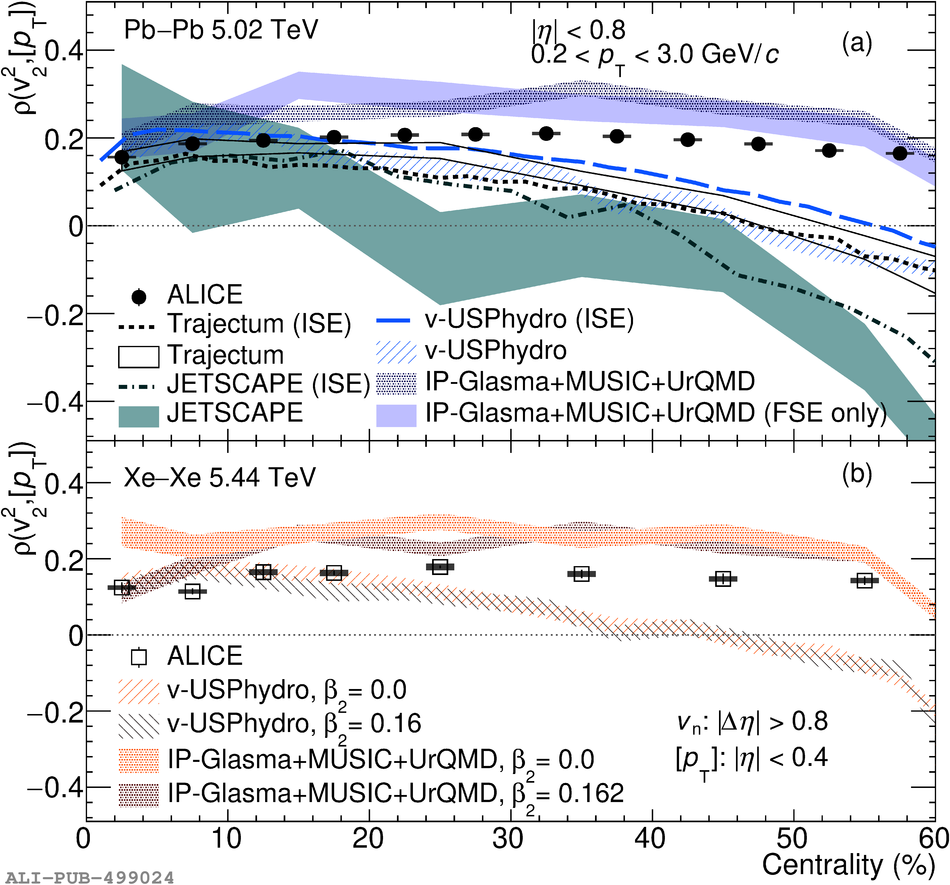Figure 1

 Centrality dependence of $\rho \left(v_{\rm 2}^{2}, [ p_{\rm T} ] \right)$ (left) and $\rho \left(v_{\rm 3}^{2}, [ p_{\rm T} ] \right)$ (right) in Pb--Pb at $\sqrt{s_{\rm NN}} = 5.02$~TeV (a, c) and Xe--Xe collisions $\sqrt{s_{\rm NN}} = 5.44$~TeV (b, d). The statistical (systematic) uncertainties are shown as vertical bars (filled boxes). The initial-state estimations (ISE) are represented by lines, while IP-Glasma+MUSIC+UrQMD~, v-USPhydro~, Trajectum~, and JETSCAPE~ hydrodynamic model calculations are shown with hatched bands.